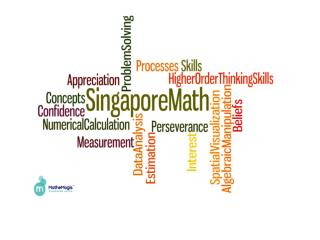# Introduction to Singapore Math - PowerPoint PPT PresentationDownload PresentationIntroduction to Singapore Math

Introduction to Singapore Math
Download Presentation## Introduction to Singapore Math

- - - - - - - - - - - - - - - - - - - - - - - - - - - E N D - - - - - - - - - - - - - - - - - - - - - - - - - - -
##### Presentation Transcript

1. Introduction to Singapore Math Back to School Night 2013 Lauren Quinn K-5 Math Coordinator

2. Why Singapore Math? • Trends in International Mathematics and Science (TIMSS) Study • Promotes deeper understanding of essential math concepts • Enhances problem-solving skills • Helps all students • As intervention strategies to support struggling learners • As extension strategies to challenge advanced learners

3. Introduction to Singapore Math C-P-A approach (Concrete-Pictorial-Abstract)

4. Warm-Up: Which combinations of 3 different whole numbers (greater than zero) have a sum of 10?We can solve this using manipulatives (concrete), model drawing (pictorial), and logic (abstract).

5. Warm-Up: Which combinations of 3 different whole numbers (greater than zero) have a sum of 10?Note: There are 4 different correct answers.

6. Introduction to Singapore Math Guided Release of Responsibility Model • Teacher Modeling • Students practice with the teacher • Small Groups/Partner • Independent Practice *Opportunities for children to explain and justify their work promotes a much higher level of thinking than just “getting the answer.”

7. Introduction to Singapore Math Number Bonds Whole/Part/Part Thinking

8. Introduction to Singapore Math Strategy: Compensation in addition 8 + 5 2 3 Think: 10 + 3 = 13

9. More Addition: Mental Math 58 + 16 27 + 56 2 14 3 53 60 + 14 = 74 30 + 53 = 83

10. More Addition: Mental Math Try It! 68 + 14 2 12 70 + 12 = 82

11. Introduction to Singapore Math 14 oz. + 6 oz. 2 4 16 oz = 1 lb. 4 oz.

12. Introduction to Singapore Math Compensation in subtraction 5,000 4,999 -3,267 -3,266 1,733

13. More Subtraction: Mental MathTry It! 90 – 18 50 – 28 2 70 20 70 + 2 = 72 90 – 63 70 - 59

14. Introduction to Singapore Math Model Drawing Carla and Jerome kept track of the miles they ran over the weekend. Carla ran three times as far as Jerome. If Carla ran 6 miles, how far did Jerome run? Carla Jerome Jerome ran ___________ miles.

15. “Singapore Math gives students a solid math foundation upon which to build increasingly complex skills, and makes it less likely that they will have to be retaught the same thing in later years.”New York Times Friday, October 1, 2010

16. “We cannot always build the future for our youth, but we can build our youth for the future.” -Franklin D. Roosevelt